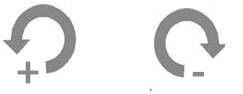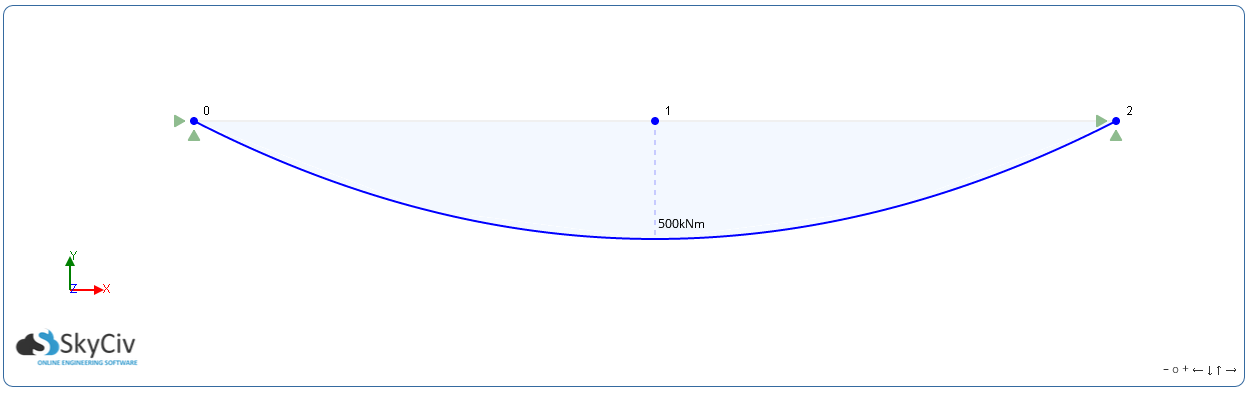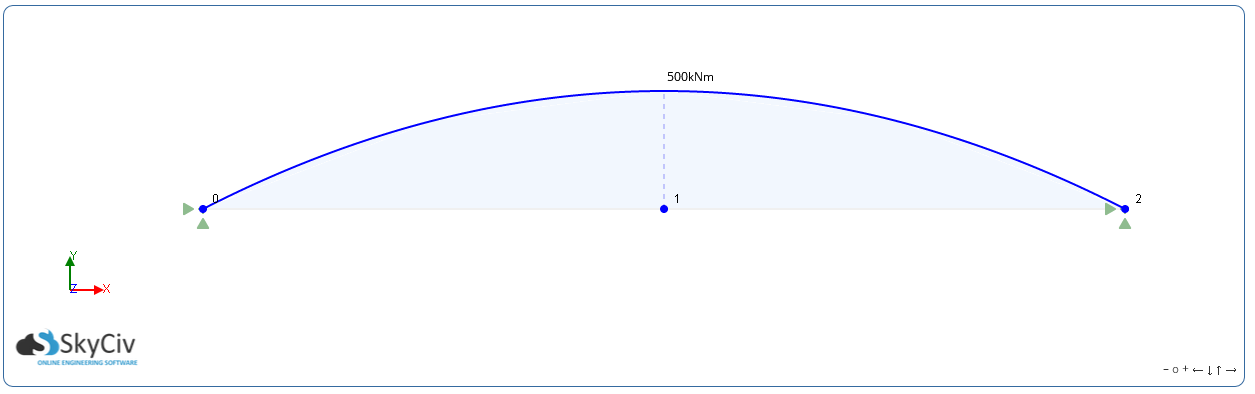# Bending Moment Convention

Ever wondered why bending moment diagrams seem inverted? Or why positive bending moments are denoted by counterclockwise arrows. If you've ever been troubled by which direction to draw or represent your result, this article should help to clear up those questions you have.

## What is the conventional direction of bending moment arrows?

It is considered convention to have a positive bending moment denoted as an anti-clockwise arrow and a negative bending moment as a clockwise arrow. It is easy to remember if you recognise that it is counterintuitive to what you would expect.## Is positive up or down in a bending moment diagram?

Let me explain this further. Bending Moment Diagrams are used to represent the bending moment forces that act along the length of a beam. Most of the time, the beam undergoes forces in the negative Y direction due to gravity. This generally induces a positive bending moment as the beam will sag or drop.

The first case: a positive bending moment shows as negative on the diagram. Sometime the graph is represented in this way to interpret the results logically; a downward force on a member will cause a sag and therefore the diagram should show this:Although this is not technically correct, it is sometimes considered as convention. It displays the result in a logical and easy to understand manner - which is why it is so commonly used. You will notice that the result is still shown as a positive 500 kNm - this is the most important value. No matter which way the force is represented, the actual magnitude of the moment is most important.

On the other hand, positive is normally denoted as being up and negative is down. There for it would also be correct to show the diagram the following way:This would be as a user expects; the +500kNm bending moment force is interpretated as being positive to the member.

Although it is not totally important which method you use, it is important that you understand both methods. You may be given design drawings that have the bending moment diagrams inverted and you need to be prepared for this in order to produce safe design practices. SkyCiv Structural, our online structural analysis software allows the bending moment diagrams to be flipped and is a very useful tool.Sam Carigliano, PEng
CEO and Co-Founder of SkyCiv
BEng (Civil), BCom